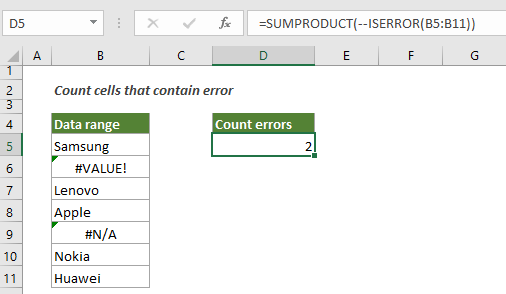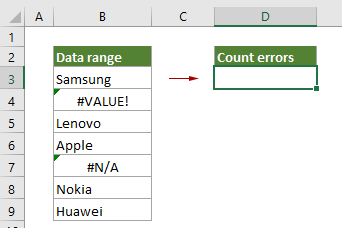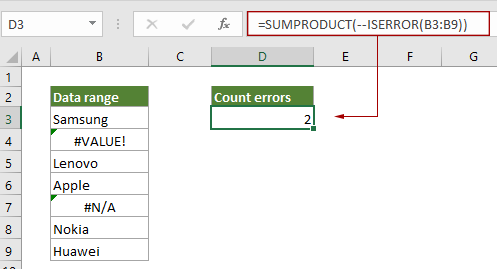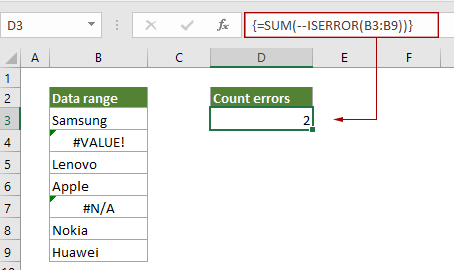## Count how many cells contain errors in a range in Excel

This tutorial shows how to count the number of cells that contains errors (any kind of errors, such as #N/A error, #VALUE! error or #DIV/0! error) in a specified range in Excel.#### Count cells contain errors with SUMPRODUCT function

As the below screenshot shown, to count how many cells contain errors in B3:B9, you can apply the SUMPRODUCT function along with the ISERR function wrapped in.Generic Formula

=SUMPRODUCT(--ISERROR(range))

Arguments

Range (required): The range from which you want to count cells that contain errors.

How to use this formula?

1. Select a blank cell such as D3 in this case.

2. Enter the below formula into it and press the Enter key to get the result.

=SUMPRODUCT(--ISERROR(B3:B9))How these formulas work?

=SUMPRODUCT(--ISERROR(B3:B9))

• 1. ISERROR(B3:B9): The ISERROR function returns TRUE when a cell in range B3:B9 contains an error, and returns FALSE if not. It finally returns an array {FALSE;TURE;FALSE;FALSE;TURE;FALSE;FALSE} which means that only the second and the fifth cell contain error values in the range.
• 2. --{FALSE;TURE;FALSE;FALSE;TURE;FALSE;FALSE}: These two minus signs convert “TRUE” into 1 and convert “FALSE” into 0. Here you will get a new array as {0;1;0;0;1;0;0}.
• 3. SUMPRODUCT{0;1;0;0;1;0;0}: The SUMPRODUCT function sums all number in the array and returns the final result as 2.

#### Count cells contain errors with SUM function

The SUM function can also help to count error cells in a range, you can do as follows.

Generic Formula

{=SUM(--ISERROR(range))}

Arguments

Range (required): The range from which you want to count cells that contain errors.

Note: This formula must be entered as an array formula. After applying the formula, if there are curly brackets wrapped around the formula, it means that an array formula is successfully created.

How to use this formula?

1. Select a blank cell such as D3 in this case.

2. Enter the below formula into it, and then press the Ctrl + Shift + Enter keys together to get the result.

=SUM(--ISERROR(B3:B9))How these formulas work?

=SUM(--ISERROR(B3:B9))

• 1. ISERROR(B3:B9): The ISERROR function returns TRUE when a cell in range B3:B9 contains an error, and returns FALSE if not. It finally returns an array {FALSE;TURE;FALSE;FALSE;TURE;FALSE;FALSE} which means that only the second and the fifth cell contain error values in the range.
• 2. --{FALSE;TURE;FALSE;FALSE;TURE;FALSE;FALSE}: These two minus signs convert “TRUE” into 1 and convert “FALSE” into 0. Here you will get a new array as {0;1;0;0;1;0;0}.
• 3. SUM{0;1;0;0;1;0;0}: The SUM function sums all number in the array and returns the final result as 2.

#### Related functions

Excel SUMPRODUCT function
The Excel COUNTBLANK function can be used to multiply two or more columns or arrays together, and then get the sum of products.

Excel ISERROR function
The ISERROR function checks whether a value is an error and returns TRUE or FALSE.

Excel SUM function

#### Related formulas

Count Blank/Nonblank Cells
This article explains formulas to count the number of blank and nonblank cells in a range in Excel.

Count cells that contain either x or y
This tutorial is going to show you how to apply a formula based on the SUMPRODUCT function in details to count the number of cells in a specific range that contain either x or y in Excel.

Count cells that contain specific text with case sensitive
This tutorial provides a formula in details to count the number of cells that contains specific text with case sensitive in a range in Excel.

Count Cells That Begin Or End With Specific Text

### The Best Office Productivity Tools

#### Kutools for Excel - Helps You To Stand Out From Crowd

 Popular Features: Find/Highlight/Identify Duplicates  |  Delete Blank Rows  |  Combine Columns or Cells without Losing Data  |  Round without Formula ... Super VLookup: Multiple Criteria  |  Multiple Value   |  Across Multi-Sheets  |  Fuzzy Lookup... Adv. Drop-down List: Easy Drop Down List  |  Dependent Drop Down List  |  Multi-select Drop Down List... Column Manager: Add a Specific Number of Columns  |  Move Columns  |  Unhide Columns  |  Compare Columns to Select Same & Different Cells ... Featured Features: Grid Focus  |  Design View  |  Big Formula Bar  |  Workbook & Sheet Manager  |  Resource Library (Auto Text)  |  Date Picker  |  Combine Worksheets  |  Encrypt/Decrypt Cells  |  Send Emails by List  |  Super Filter  |  Special Filter (filter bold/italic/strikethrough...) ... Top 15 Toolsets:  12 Text Tools (Add Text, Remove Characters ...)  |  50+ Chart Types (Gantt Chart ...)  |  40+ Practical Formulas (Calculate age based on birthday ...)  |  19 Insertion Tools (Insert QR Code, Insert Picture from Path ...)  |  12 Conversion Tools (Numbers to Words, Currency Conversion ...)  |  7 Merge & Split Tools (Advanced Combine Rows, Split Cells ...)  |  ... and more

Kutools for Excel Boasts Over 300 Features, Ensuring That What You Need is Just A Click Away...

Supports Office/Excel 2007-2021 and 365   |   Available in 44 Languages   |   Easy to Uninstall Completely#### Office Tab - Enable Tabbed Reading and Editing in Microsoft Office (include Excel)

• One second to switch between dozens of open documents!
• Reduce hundreds of mouse clicks for you every day, say goodbye to mouse hand.
• Increases your productivity by 50% when viewing and editing multiple documents.
• Brings Efficient Tabs to Office (include Excel), Just Like Chrome, Edge and Firefox.